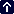whiz-wheel

 Wind Speed Result can be either Knots or MPH based on consistent entry of the same designation. Enter   Ground Speed Enter   True Airspeed Enter   Course Enter   Heading Calculated Wind Speed =
 Wind Direction Result can be either Knots or MPH based on consistent entry of the same designation. Enter   Ground Speed Enter   True Airspeed Enter   Course Enter   Heading Calculated Wind Direction =
 Heading, Ground Speed, And Wind Correction Angle Result can be either Knots or MPH based on consistent entry of the same designation. Enter   Wind Speed Enter   Wind Direction Enter   True Airspeed Enter   Course Calculated   Heading Calculated   Ground Speed Calculated   Wind Correction AngleCourse, Ground Speed, And Wind Correction Angle Result can be either Knots or MPH based on consistent entry of the same designation. Enter   Wind Speed Enter   Wind Direction Enter   True Airspeed Enter   Heading Calculated   Course Calculated   Ground Speed Calculated   Wind Correction Angle
 Density Altitude Enter   Pressure Altitude (Ft) Enter   Actual Temperature F° C° Calculated Density Altitude =Runway Crosswinds
 Runway (i.e. 220) Wind Direction (i.e. 270) Wind Speed Knots The Crosswind is Knots
 Fahrenheit to Celsius  This conversion of temperature is made using the formula (Fahrenheit temperature * 9/5) + 32) Enter   °F Calculated Temperature = °CCelsius to Fahrenheit  This conversion of temperature is made using the formula (5/9 * (Celsius temperature - 32)) Enter   °C Calculated Temperature = °F
 Wind Chill  Wind chill cannot be accurately calculated for outside air temperatures (OAT) greater that 50 °F (10 °C) and wind speeds less than 4 MPH (3.5 Knots). Enter   Temperature (OAT) °F °C Enter   Wind MPH Knots Calculated Wind Chill = °F or °C
 Heat Index  Determine the heat index from the outside air temperature (OAT) and Relative Humidity. Enter   Temperature (OAT) °F °C Enter   % Relative Humidity Calculated Heat Index = °F or °C
 Dewpoint  Calculate the Dewpoint from the outside air temperature (OAT) and Relative Humidity Enter   Temperature (OAT) °F °C Enter   % Relative Humidity Calculated Dewpoint = °F °C
 Relative Humidity Calculate the Relative Humidity from the outside air temperature (OAT) and Dewpoint Enter   Temperature (OAT) °F °C Enter   Dewpoint °F °C Calculated Relative Humidity =  %
 Pressure Conversion Convert Millibars to Inches of Mercury and Inches of Mercury to Millibars Enter   Millibars Inches Mercury Converted Barometric Pressure =
 Miles Per Hour To Knots  This conversion of speed (or distance) is made using the formula (Knots (or nautical miles) = MPH (or miles) * 0.86897624) Enter   MPH or Statute Miles Calculated Knots Or Nautical Miles =
 Knots to Miles Per Hour  This conversion of speed (or distance) is made using the formula (MPH (or statute miles) = Knots (or nautical miles) * 1.15077945) Enter   Knots or Nautical Miles Calculated MPH or Statute Miles =
 True Airspeed (TAS or KTAS) Result can be either Knots or MPH based on consistent entry of the same designation. The formula is (IAS) + (.02 x MSL / 1000). Enter   Indicated Airspeed Enter   MSL Altitude Calculated True Airpeed =